# Boyles Law What is Boyles Law Boyles Law

• Slides: 14Boyle’s Law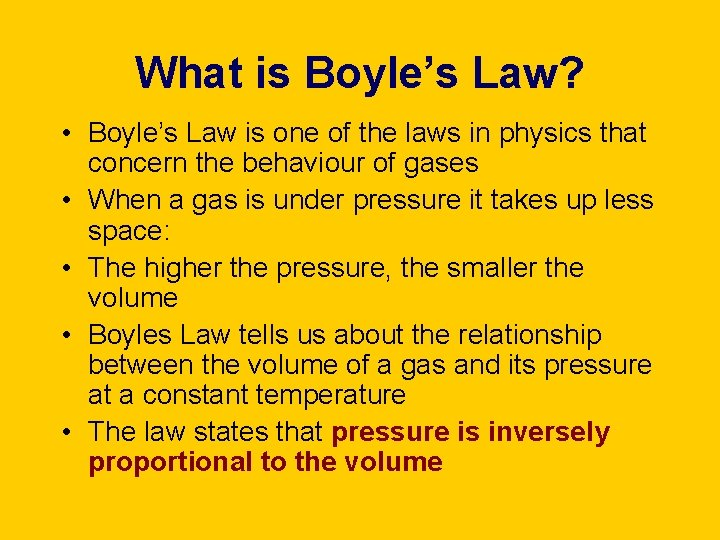What is Boyle’s Law? • Boyle’s Law is one of the laws in physics that concern the behaviour of gases • When a gas is under pressure it takes up less space: • The higher the pressure, the smaller the volume • Boyles Law tells us about the relationship between the volume of a gas and its pressure at a constant temperature • The law states that pressure is inversely proportional to the volumeHow can we write Boyle’s Law as a formula? • Pressure is inversely proportional to the volume and can be written as: • Pressure a 1/volume P=pressure in N/m 2 V=volume in dm 3 (litres) k=constant • This is more usually written as: • Pressure = constant volume • PV=k • P 1 V 1=P 2 V 2How can we investigate Boyle’s Law? • When investigating Boyles law a given volume of gas is sucked into a cylinder and the end is sealed • The temperature of the gas is kept constant • Using several equal weights we can apply increasing pressure to the gas • We can calculate the pressure by dividing the force applied by the area of the top of the cylinder • The volume will be shown on the scale on the cylinder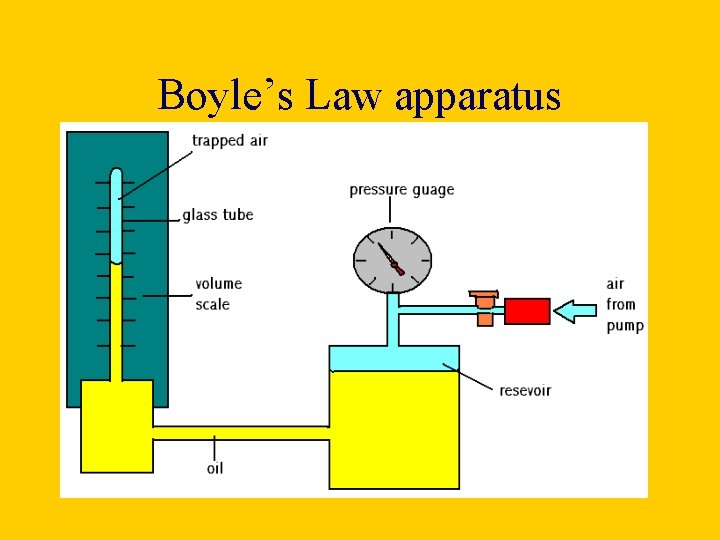Boyle’s Law apparatus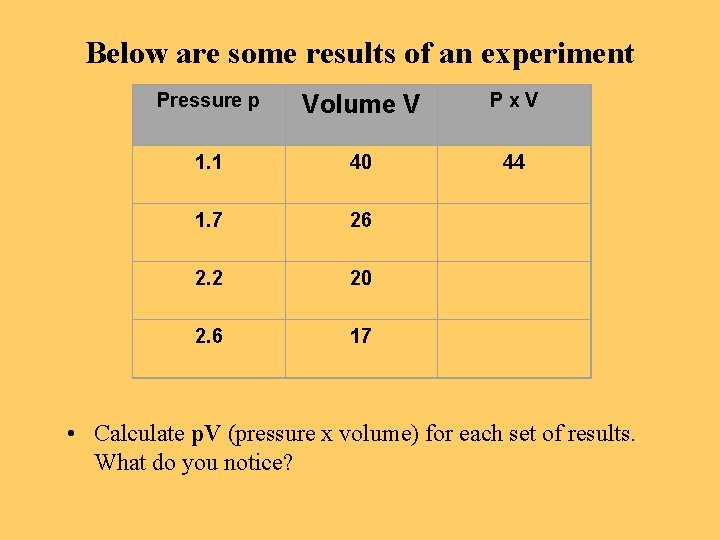Below are some results of an experiment Pressure p Volume V Px. V 1. 1 40 44 1. 7 26 2. 2 20 2. 6 17 • Calculate p. V (pressure x volume) for each set of results. What do you notice?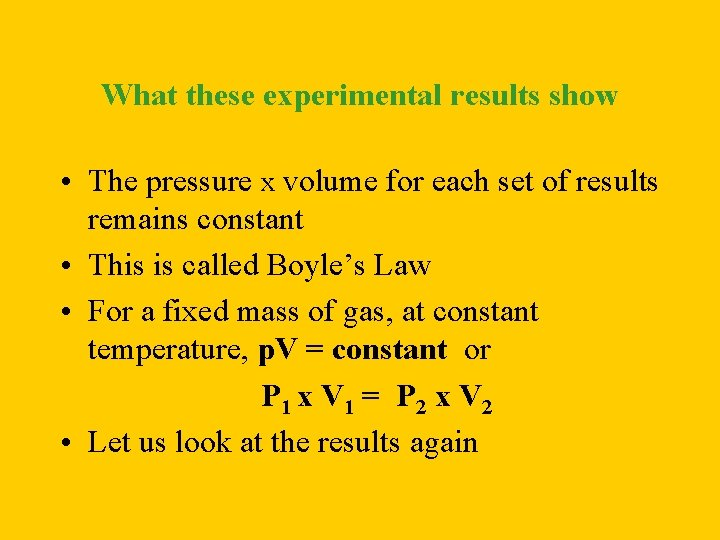What these experimental results show • The pressure x volume for each set of results remains constant • This is called Boyle’s Law • For a fixed mass of gas, at constant temperature, p. V = constant or P 1 x V 1 = P 2 x V 2 • Let us look at the results again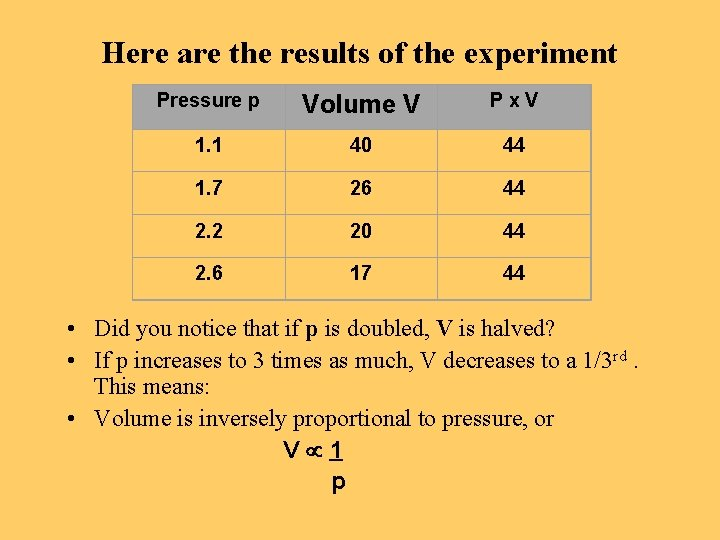Here are the results of the experiment Pressure p Volume V Px. V 1. 1 40 44 1. 7 26 44 2. 2 20 44 2. 6 17 44 • Did you notice that if p is doubled, V is halved? • If p increases to 3 times as much, V decreases to a 1/3 rd. This means: • Volume is inversely proportional to pressure, or V 1 p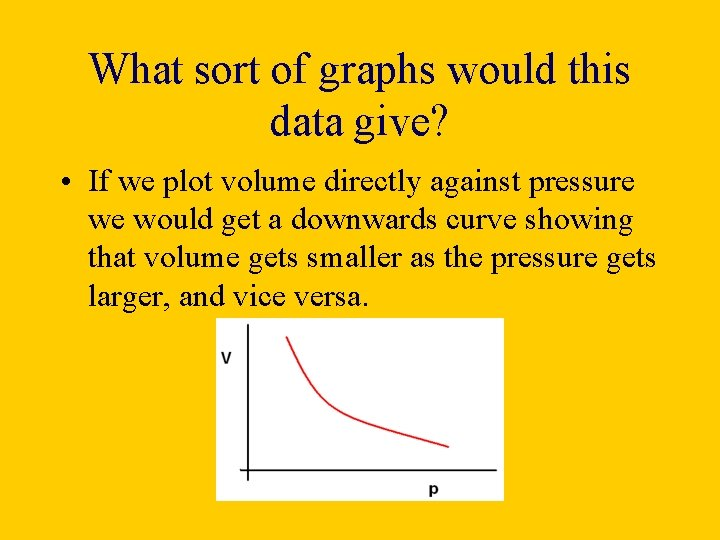What sort of graphs would this data give? • If we plot volume directly against pressure we would get a downwards curve showing that volume gets smaller as the pressure gets larger, and vice versa.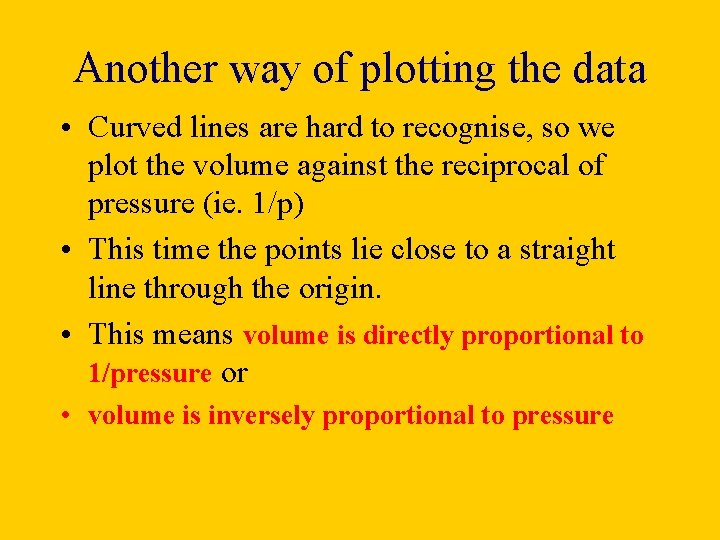Another way of plotting the data • Curved lines are hard to recognise, so we plot the volume against the reciprocal of pressure (ie. 1/p) • This time the points lie close to a straight line through the origin. • This means volume is directly proportional to 1/pressure or • volume is inversely proportional to pressureThis leads us back to Boyle’s Law: for a fixed mass of gas kept at constant temperature the volume of the gas is inversely proportional to its pressure.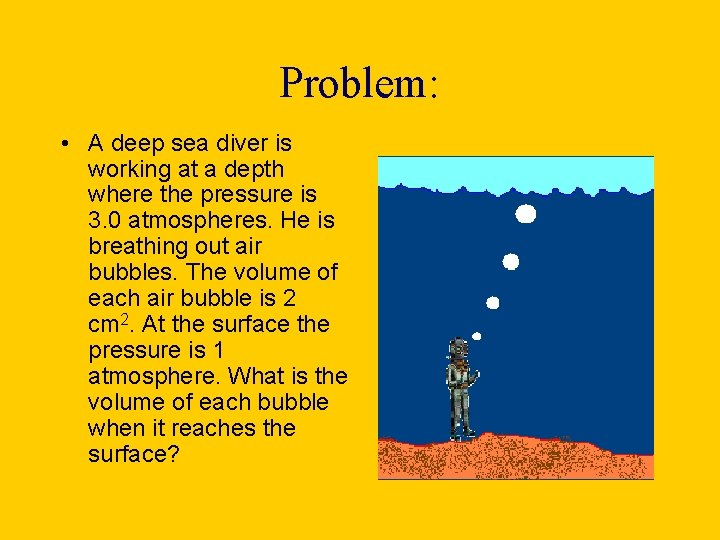Problem: • A deep sea diver is working at a depth where the pressure is 3. 0 atmospheres. He is breathing out air bubbles. The volume of each air bubble is 2 cm 2. At the surface the pressure is 1 atmosphere. What is the volume of each bubble when it reaches the surface?How we work this out: • We assume that the temperature is constant, so Boyle’s Law applies: • Formula first: P 1 x V 1 = P 2 x V 2 • Then numbers: = 1. 0 x 2 = 3. 0 x V 2 • Now rearrange the numbers so that you have V 2 on one side, and the rest of the numbers on the other side of the ‘equals’ symbol.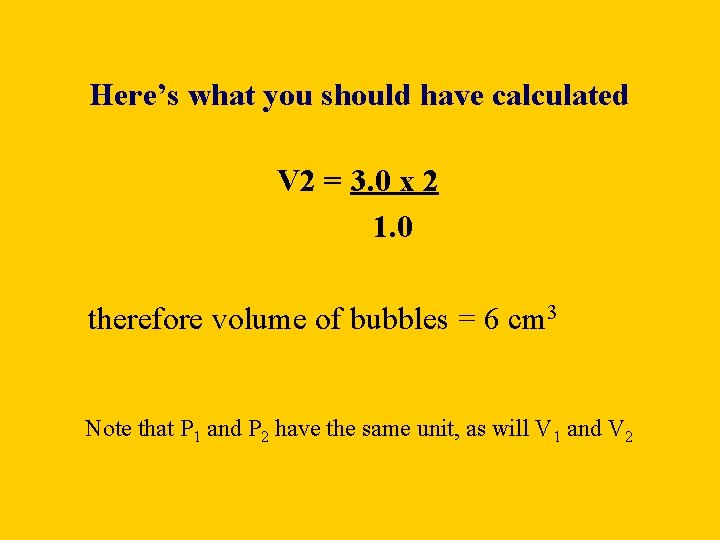Here’s what you should have calculated V 2 = 3. 0 x 2 1. 0 therefore volume of bubbles = 6 cm 3 Note that P 1 and P 2 have the same unit, as will V 1 and V 2